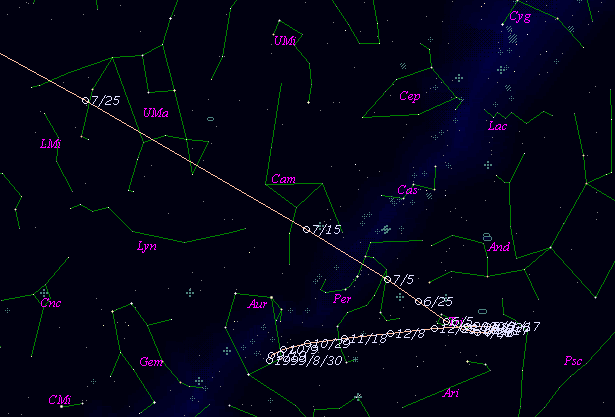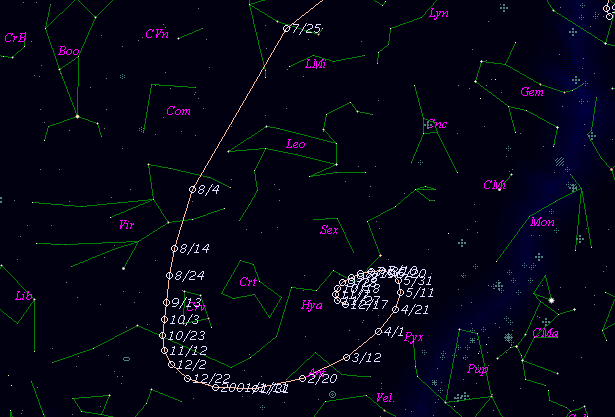# C/1999 S4 ( LINEAR )###Profile

 Discovery Date September 27, 1999 Magnitude 17.3 mag Discoverer Lincoln Laboratory Near-Earth Asteroid Research project

###Pictures###Orbital Elements

```   The following improved orbital elements by Kenji Muraoka, are
from 776 observations 1999 Sept. 27 to 2000 June 1, perturbations
by 9 Planets, Moon and 5 minor planets were taken into account.
The mean residual is +/- 0.64 arc seconds.

Epoch  =  2000 Aug.  4.0  TT       JDT = 2451760.5
T  =  2000 July 26.16847       +/- 0.00071 (m.e.) TT
Peri. =  151.06718                +/- 0.00017
Node  =   83.19042                +/- 0.00022   (2000.0)
Incl. =  149.38993                +/- 0.00009
q  =    0.7650068              +/- 0.0000065 AU
e  =    0.9999970              +/- 0.0000016
1/a  =   +0.0000039              +/- 0.0000020 1/AU
orig. 1/a  =   +0.0000029
fut.  1/a  =   +0.0010393
```

###Finding Charts###Magnitudes Graph

```        m1 =  8.3 + 5 log d +   8.0 log r  [   ,-55]  (             - 2000 June  1)
m1 =  7.6 + 5 log d +  15   log r  [-55,-35]  (2000 June  1 - 2000 June 21)
m1 =  7.7 + 5 log d -  10   log r  [-35, -5]  (2000 June 21 - 2000 July 21)
m1 = 20.1 + 5 log d + 100   log r  [ -5,  0]  (2000 July 21 - 2000 July 26)
m1 = 46.3 + 5 log d + 325   log r  [  0, 13]  (2000 July 26 - 2000 Aug.  8)
m1 = 17   + 5 log d +  15   log r  [ 13,   ]  (2000 Aug.  8  -            )

* Gray curve is:  m1 = 8.3 + 5 log d + 8.0 log r
```##### The orbital elements are calculated by Kenji Muraoka. The charts are made with StellaNavigator Ver.2.0 for Windows (AstroArts / ASCII). The magnitudes graphs are made with Comet for Windows.